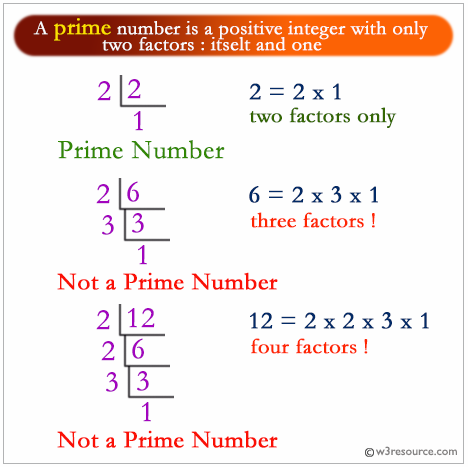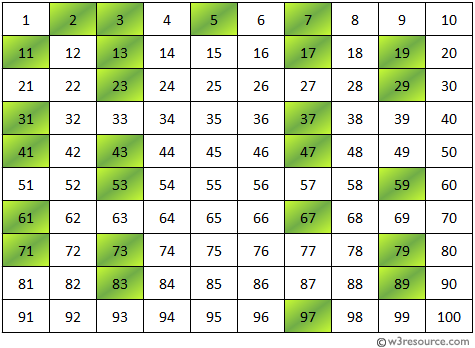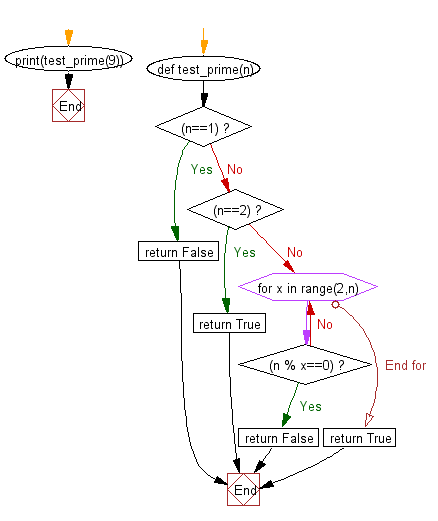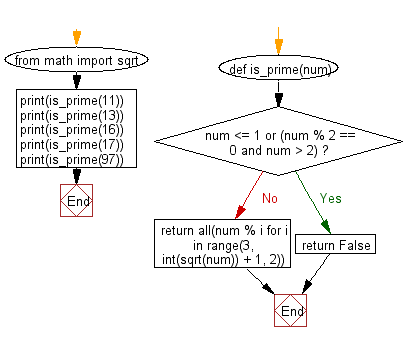﻿ Python Exercise: Test the number is prime or not - w3resource# Python Exercise: Test the number is prime or not

## Python Functions: Exercise-9 with Solution

Write a Python function that takes a number as a parameter and check the number is prime or not.

Note : A prime number (or a prime) is a natural number greater than 1 and that has no positive divisors other than 1 and itself.Prime number between 1 to 100:Sample Solution-1:

Python Code:

``````def test_prime(n):
if (n==1):
return False
elif (n==2):
return True;
else:
for x in range(2,n):
if(n % x==0):
return False
return True
print(test_prime(9))
```
```

Sample Output:

```False
```

Flowchart:## Visualize Python code execution:

The following tool visualize what the computer is doing step-by-step as it executes the said program:

Sample Solution-2:

• Return False if the number is 0, 1, a negative number or a multiple of 2.
• Use all() and range() to check numbers from 3 to the square root of the given number.
• Return True if none divides the given number, False otherwise.

Python Code:

``````from math import sqrt
def is_prime(num):
if num <= 1 or (num % 2 == 0 and num > 2):
return False
return all(num % i for i in range(3, int(sqrt(num)) + 1, 2))
print(is_prime(11))
print(is_prime(13))
print(is_prime(16))
print(is_prime(17))
print(is_prime(97))
```
```

Sample Output:

```True
True
False
True
True
```

Flowchart:## Visualize Python code execution:

The following tool visualize what the computer is doing step-by-step as it executes the said program:

Python Code Editor:

Have another way to solve this solution? Contribute your code (and comments) through Disqus.

What is the difficulty level of this exercise?

Test your Programming skills with w3resource's quiz.

﻿

## Python: Tips of the Day

Decapitalizes the first letter of a string:

Example:

```def tips_decapitalize(s, upper_rest=False):
return s[:1].lower() + (s[1:].upper() if upper_rest else s[1:])
print(tips_decapitalize('PythonTips'))
print(tips_decapitalize('PythonTips', True))
```

Output:

```pythonTips
pYTHONTIPS
```